### 3.3Time Dependent Problems

Time dependence analysis is not particularly interesting for elastic deformations, since the material recovers entirely after load removal. However, for plastic deformations, the duration of a force application defines the amount of permanent deformation within the body. Actually, plasticity has an elegant treatment with the FEM, which has been developed along the years . Although plastic deformation is studied in this work, the classical approach of plasticity is not used. Instead, a specific model based on ordinary differential equations (ODE) is employed as will be discussed in Chapter 5. This section intends to provide the background for the treatment of these kinds of equations. Time dependent problems can be presented in the general form by(3.37)

These types of equations, where the solution to the first step (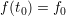) is known, are defined as initial value problems (IVP). High order ODEs can always be converted to a system of N first order equations in the same form as (3.37) by substitution. For example, the ODE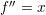can be rewritten as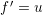and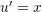.

In general, the strategy for solving (3.37) numerically consists of the division of the domain into small time steps; and for each step the solution is computed as shown in Fig. 3.9.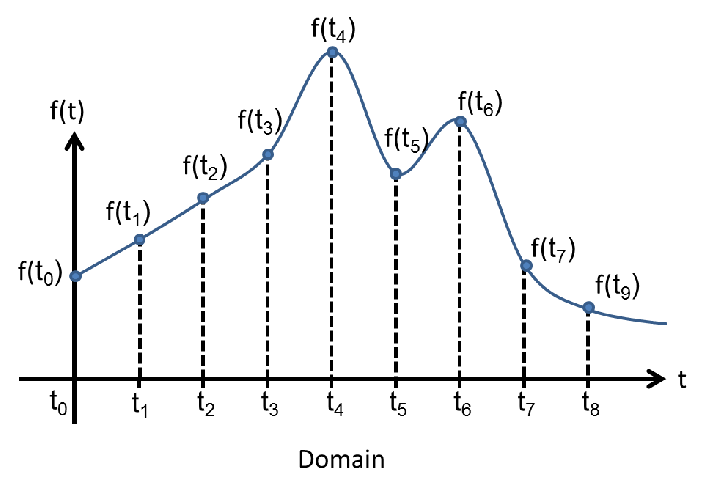Figure 3.9.: Partioning of the domain in time steps. The solution is computed at each time step.

Numerical methods for ODEs can be divided between explicit and implicit methods. The former handles (3.37) by computing the next state of the system based solely on the current state (), while implicit methods require also the future state (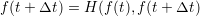). The formulation of implicit methods seems rather paradoxical, but in practice only an estimation of the future state is used to compute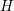. Explicit methods are more intuitive and easy to implement, but they require an unreasonable number of steps to properly approximate problems with fast variations, the so called stiff problems, for which implicit methods are more suitable.

In order to exemplify both categories, consider the approximations for the derivatives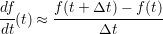(3.38)

and(3.39)

Substituting (3.38) and (3.39) in (3.37) the relations hold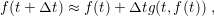(3.40)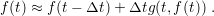(3.41)

One can notice the particular difference between the representation of (3.40) and (3.41). For the first case the unknown variable (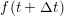) is defined explicitly by the terms on the right hand side. In the second case the unknown (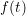) is needed in order to compute the function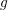, which leads to an implicit definition of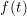. Each equation defines a method for the solution of (3.37), where (3.40) is known as the Euler method and (3.41) as backward Euler method.

In the scope of this work, implicit methods are more relevant, mainly because of exponential variations in the models for describing plasticity in Chapter 5. The so called Back Differentiation Formula (BDF) is the employed method for ODEs. The BDF method approximates the function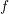by a Lagrangian polynomial as defined by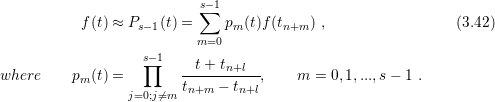The meaning ofwill become clear soon, but right now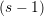can be understood as the degree of the Lagrange polynomial. From the polynomial definition (3.42) the BDF method can be built. The derivative ofis approximated by the derivative of the Lagrangian polynomial as described by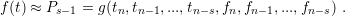(3.43)

From (3.43) one can see thatdefines the number of past states, which is used to calculate the current state of the system. Asincreases the local error of the method reduces, but for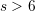the BDF is no longer convergent. Naturally, higher values ofimply higher computational demands; hence a compromise between speed and accuracy must be met. Table 3.1 represents the BDF method after expansion of the Lagrangian polynomial for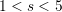.

 Table 3.1.: Expansion of the Lagrangian polynomial for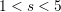.
 Order Expression BDF 1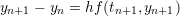BDF 2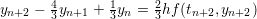BDF 3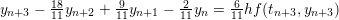BDF 4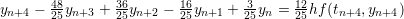BDF 5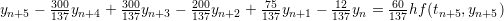In practice, the computation for each time step requires the solution of a non-linear equation. Newton’s method is the most common choice for solving the non-linear problem, but other methods can be more suitable, depending on the form of. As an initial guess, an explicit method (such as the Euler method) can be used to provide a reasonable choice for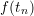.

Naturally, the effort to compute the solution at each time step is bigger for BDF methods than for explicit methods, but the argument for their utilization lies on the stability. Implicit methods are more stable than explicit methods, an important feature for the numerical solution of stiff ODEs. Such problems are by definition numerically unstable, which means that small deviations of the solution in a particular step lead to a large error in the subsequent steps. In fact, it can be proved that for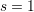, the BDF is A-stable . This means that for an ODE in the form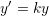, the exact solution (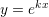) and the BDF solution are asymptotically equivalent for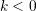. Such a condition is only valid in the Euler method for very small time steps . Evidently,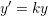is not a general case, but it is commonly used as a test problem to evaluate the stability of numerical methods for ODEs.